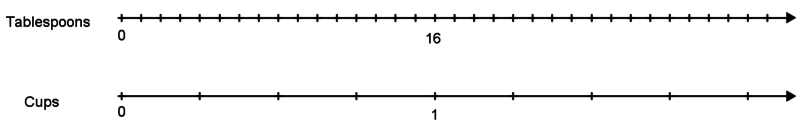# Simple Unit Conversion Using Ratio Reasoning

Alignments to Content Standards: 6.RP.A.3.d

Malik is using a cookbook to make a recipe, but he can not find his measuring cups! He has, however, found a tablespoon. Inside the back cover of the cookbook, it says that 1 cup = 16 tablespoons.

1. Explain how he could use the tablespoon to measure out the following ingredients:
1. 2 cups of flour
2. ½ cup sunflower seeds
3. 1¼ cup of oatmeal
2. Malik also adds the following ingredients. How many cups of each did he add?
1. 28 tablespoons of sugar
2. 6 tablespoons of cocoa powder

Use of the following representations is optional:Tablespoons 16 Cups 1

## IM Commentary

The purpose of this instructional task is for students to use rate and ratio reasoning to solve unit conversion problems. In grade 6, unit conversion should be approached as a case of ratio reasoning, rather than a separate procedure to learn, and this task is an example of what that might look like. This task should come after students have spent time building up their understanding of equivalent ratios and are comfortable with some different representations of equivalent ratios.

The task could be made more challenging in MP5, selecting and using appropriate tools, by taking away the representation templates (the double number line and the table), and giving students the opportunity to create them on their own.

To help students who might struggle with MP2, reasoning abstractly and quantitatively, a teacher might provide some concrete measuring tools for experimentation:

• cup measures of different sizes
• tablespoon measure
• a container of a substance that can be poured (like water with food coloring or dry rice)
• an empty container

## Solution

The table shows the correct unit conversions:

 Question Given a.i a.ii ai.ii a.iii b.i b.ii Tablespoons 16 32 8 4 20 28 6 Cups 1 2 $\frac12$ $\frac14$ $1\frac14$ $1\frac34$ $\frac38$
1. Given a number of tablespoons, find the number of cups:
1. Since he needs 2 cups, he can use $2 \times 16 = 32$ tablespoons.
2. Since he needs $\frac12$ cup, he can use $\frac12 \times 16 = 8$ tablespoons.
3. He needs $1\frac14$ cups. 1 cup is 16 tablespoons. $\frac14$ cup is $\frac14 \times 16 = 4$ tablespoons. So altogether he needs $16+4=20$ tablespoons.
2. Given a number of cups, find the number of tablespoons:
1. I already found that 20 tablespoons is $1\frac14$ cups. So for 28 tablespoons I need an additional 8 tablespoons, or an additional $\frac12$ cup. $1\frac14 + \frac12 = 1\frac34$, so 28 tablespoons gets him $1\frac34$ cups. (Alternatively, we might say that 1 tablespoon is $\frac{1}{16}$ cups. $\frac{1}{16} \times 28= \frac{28}{16}$, which we can rewrite as $1\frac{12}{16}$ or $1\frac34$.)
2. I notice that to convert from tablespoons to cups, I always divide by 16. $6\div16=\frac{6}{16}$, which we can write as $1\frac38$. (Alternatively, 1 tablespoon is $\frac{1}{16}$ cups. $\frac{1}{16} \times 6= \frac{6}{16}$, which we can rewrite as $1\frac38$.)